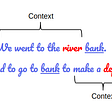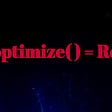# neural network programming error

clear all;clc

`a=rand(1,1000); b=rand(1,1000); c=rand(1,1000); y=a*2+b*3+c*5 I=[a; b; c;] T=y net = newff([0 1;0 1 ;0 1],[10 1]); net=train(net,I,T); J1=sim(net,I); Op=sim(net,[1 1 1]');`

As i am testing system with inputs [1 1 1] then the output must be neat to 10, but it gives 1. what is wrong with this code?

Matlabsolutions.com provide latest MatLab Homework Help,MatLab Assignment Help for students, engineers and researchers in Multiple Branches like ECE, EEE, CSE, Mechanical, Civil with 100% output.Matlab Code for B.E, B.Tech,M.E,M.Tech, Ph.D. Scholars with 100% privacy guaranteed. Get MATLAB projects with source code for your learning and research.

`clear all; clc rng('default')    % Initialized the RNG a = rand(1,1000); b = rand(1,1000); c = rand(1,1000); y = a*2+b*3+c*5;  % Added semicolon I = [a; b; c;];   % Added semicolon T = y;            % Added semicolon net = newff([0 1;0 1 ;0 1],[10 1]); net=train(net,I,T); J1=sim(net,I); MSE = mse(J1-T) Op=sim(net,[1 1 1]') %  Warning: NEWFF used in an obsolete% way. % > In obs_use at 18%   In newff>create_network at 127%   In newff at 102%   In Untitledgh at 9 %   See help for NEWFF to update calls to %   the   new argument list. % MSE =   19.6749 % Op =     1 net = newff( I ,T ,10 );  % Current version net=train(net,I,T); J1=sim(net,I); MSE = mse(J1-T)        Op=sim(net,[1 1 1]')    % MSE =   4.4650e-009 % Op  =     9.9969`

To be sure the obsolete version contains a bug,

https://www.matlabsolutions.com/resources/neural-network-programming-error.php

--

--

--

## More from Technical Source

Simple! That is me, a simple person. I am passionate about knowledge and reading. That’s why I have decided to write and share a bit of my life and thoughts to.

Love podcasts or audiobooks? Learn on the go with our new app.

## Introducing FathomNet (beta)!## Data Augmentation —  Is it really necessary?## Differences Between Word2Vec and BERT## Development of social chatbots## Robustly optimized BERT Pretraining Approaches## Random Forest Algorithm## Deep Q Learning — A Reinforcement Learning Algorithm## Technical Source

Simple! That is me, a simple person. I am passionate about knowledge and reading. That’s why I have decided to write and share a bit of my life and thoughts to.

## Fix Attribute Error and Runtime Error in tensorflow and keras | Beginner Must Read## How to stay up to date in machine learning and deep learning?## The Chain Rule of Conditional Probabilities